# Solving TwoStep Equations You will solve equations by

• Slides: 15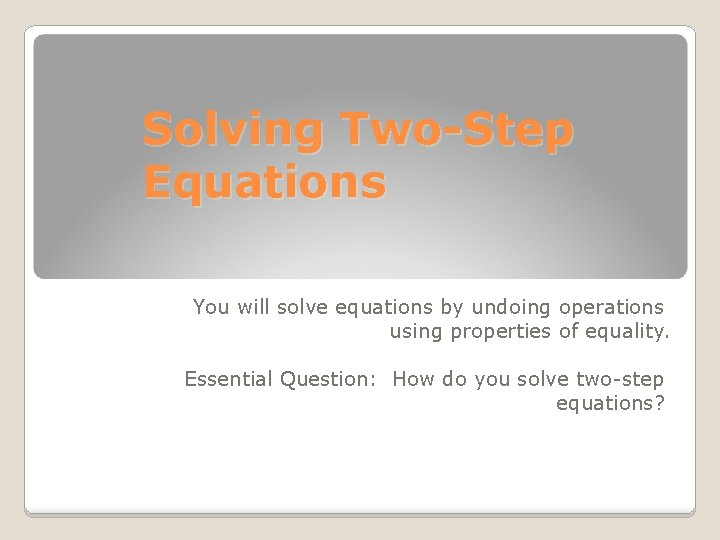Solving Two-Step Equations You will solve equations by undoing operations using properties of equality. Essential Question: How do you solve two-step equations?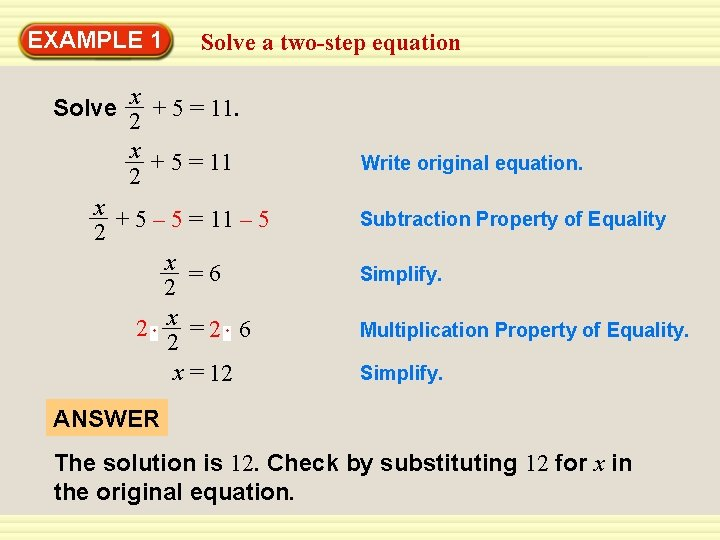EXAMPLE 1 Solve a two-step equation x + 5 = 11. 2 x + 5 = 11 2 x + 5 – 5 = 11 – 5 2 x =6 2 2 x =2 6 2 x = 12 Write original equation. Subtraction Property of Equality Simplify. Multiplication Property of Equality. Simplify. ANSWER The solution is 12. Check by substituting 12 for x in the original equation.EXAMPLE 1 CHECK Solve a two-step equation x + 5 = 11 Write original equation. 2 12 + 5 ? = 11 Substitute 12 for x. 2 11 = 11 Simplify. Solution checks.GUIDED PRACTICE for Example 1 Solve the equation. Check your solution. 1. 5 x + 9 = 24 SOLUTION 5 x + 9 = 24 5 x + 9 – 9 = 24 – 9 5 x = 15 5 x 15 5 = 5 x=3 Write original equation. Subtraction Property of Equality Simplify. Division Property of Equality Simplify.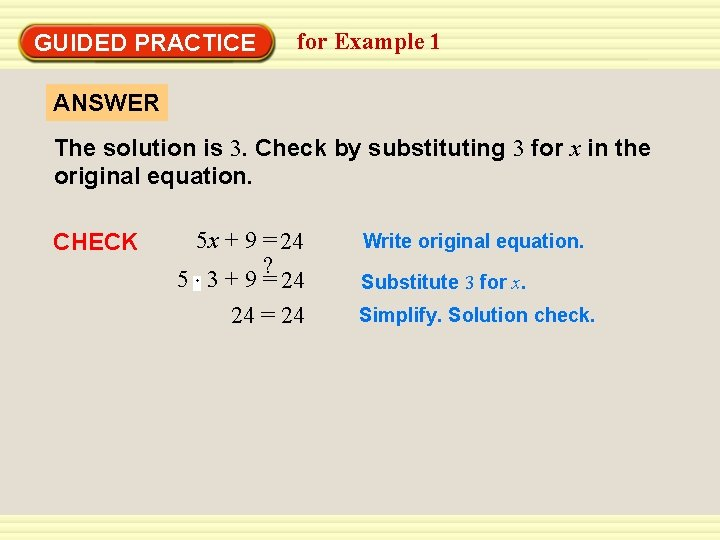GUIDED PRACTICE for Example 1 ANSWER The solution is 3. Check by substituting 3 for x in the original equation. CHECK 5 x + 9 = 24 ? 5 3 + 9 = 24 24 = 24 Write original equation. Substitute 3 for x. Simplify. Solution check.GUIDED PRACTICE for Example 1 Solve the equation. Check your solution. 2. 4 y – 4 = 16 SOLUTION 4 y – 4 = 16 4 y – 4 + 4 = 16 + 4 4 y = 20 4 y 20 = 4 4 y=5 Write original equation. Addition Property of Equality Simplify. Division Property of Equality. Simplify.GUIDED PRACTICE for Example 1 ANSWER The solution is 5. Check by substituting 5 for y in the original equation. CHECK 4 y – 4 = 16 ? 4(5) – 4 = 16 16 = 16 Write original equation. Substitute 5 for y. Simplify. Solution checks.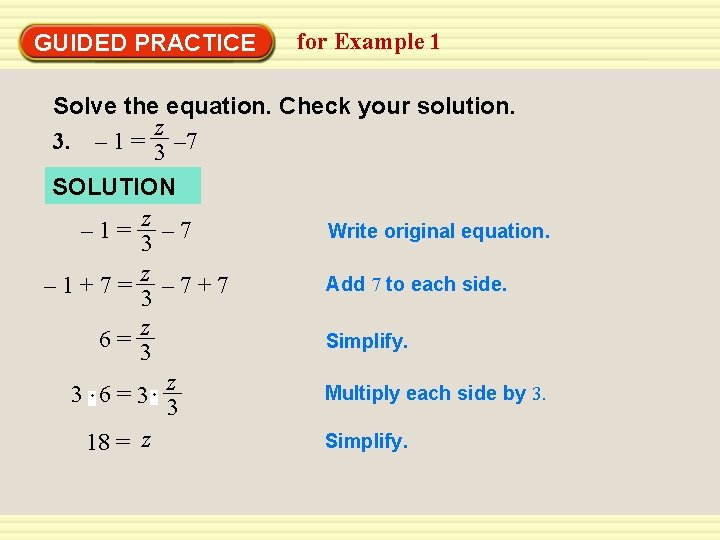GUIDED PRACTICE for Example 1 Solve the equation. Check your solution. z 3. – 1 = 3 – 7 SOLUTION – 1= z – 7 3 z – 1+7= – 7+7 3 z 6= 3 z 3 6=3 3 18 = z Write original equation. Add 7 to each side. Simplify. Multiply each side by 3. Simplify.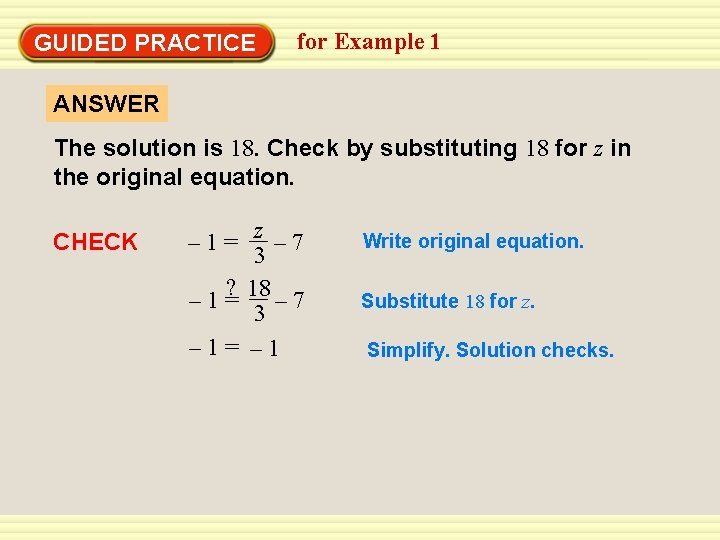GUIDED PRACTICE for Example 1 ANSWER The solution is 18. Check by substituting 18 for z in the original equation. CHECK z – 7 3 ? 18 – 1= – 7 3 – 1= Write original equation. Substitute 18 for z. Simplify. Solution checks.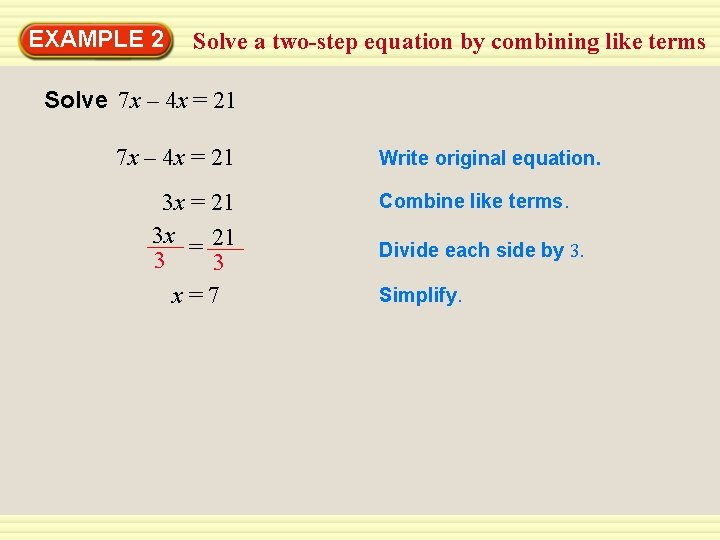EXAMPLE 2 Solve a two-step equation by combining like terms Solve 7 x – 4 x = 21 3 x = 21 3 3 x=7 Write original equation. Combine like terms. Divide each side by 3. Simplify.GUIDED PRACTICE for Examples 2 and 3 Solve the equation. Check your solution. 5. 8 t – 3 t = 35 5 t = 355 5 t =7 Write original equation. Substract the like terms Divide each side by 5 Simplify.GUIDED PRACTICE CHECK 8 t – 3 t = 35 8(7) – 3(7) =? 35 ? 56 – 21 = 35 35 =35 for Examples 2 and 3 Write original equation. Substitute 7 for t. Multiply. Simplify. Solution checks.GUIDED PRACTICE for Example 4 Jobs 10. Kim has a job where she makes \$8 per hour plus tips. Yesterday, Kim made \$53 dollars, \$13 of which was from tips. How many hours did she work? SOLUTION 53 = 13 + 8 h 53– 13 = 13 – 13 + 8 h 40 = 8 h 5=h Write the equation Subtraction Property of Equality. Simplify. Division Property of EqualityGUIDED PRACTICE for Example 4 ANSWER She worked for 5 hours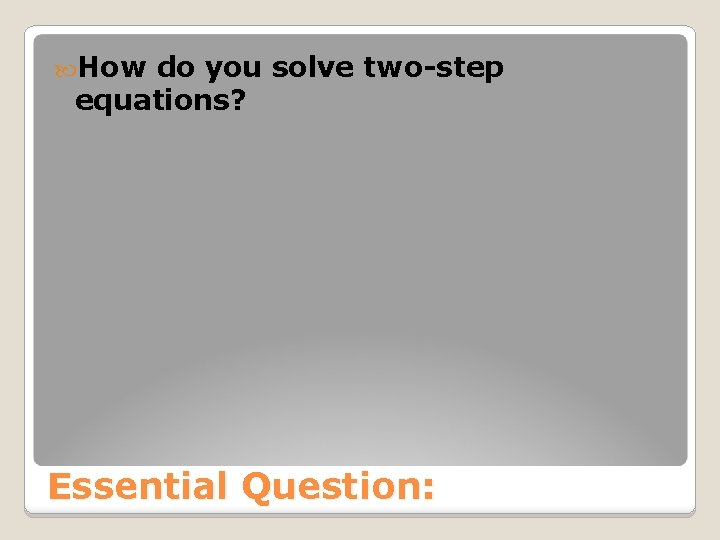How do you solve two-step equations? Essential Question: